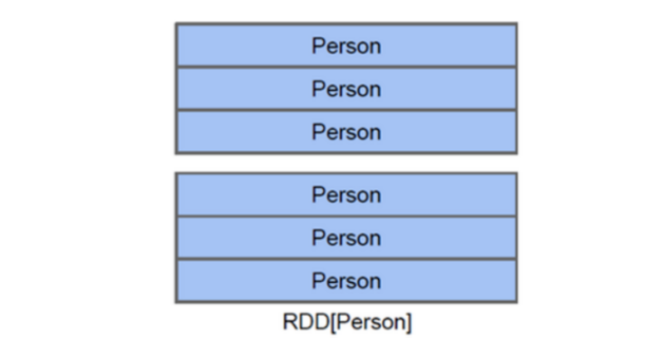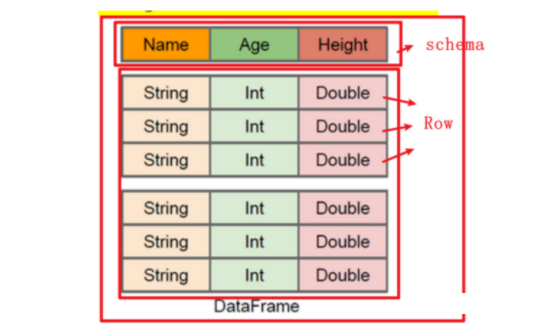# 大数据培训：RDD、DataFrame 的区别

• 2022 年 3 月 07 日
• 本文字数：3005 字

阅读完需：约 10 分钟

​RDD、DataFrame 是什么

RDD（Resilient Distributed Datasets）提供了一种高度受限的共享内存模型。

RDD 仍然足以表示很多类型的计算，包括 MapReduce 和专用的迭代编程模型（如 Pregel）等。

DataFrame 是一种分布式的数据集，并且以列的方式组合的。大数据培训类似于关系型数据库中的表。可以说是一个具有良好优化技术的关系表。

DataFrame 背后的思想是允许处理大量结构化数据。提供了一些抽象的操作，如 select、filter、aggregation、plot。

DataFrame 包含带 schema 的行。schema 是数据结构的说明。相当于具有 schema 的 RDD。

RDD、DataFrame 有什么特性

RDD 和 DataFrame 的共同特征是不可性、内存运行、弹性、分布式计算能力。

DataFrame 的应用程序编程接口(api)可以在各种语言中使用，包括 Python、Scala、Java 和 R。

RDD 的五大特性：

1.(必须的)可分区的: 每一个分区对应就是一个 Task 线程。

2.(必须的)计算函数(对每个分区进行计算操作)。

3.(必须的)存在依赖关系。

4.(可选的)对于 key-value 数据存在分区计算函数。

5.(可选的)移动数据不如移动计算(将计算程序运行在离数据越近越好)。

DataFrame 的特性：

1.支持从 KB 到 PB 级的数据量。

2.支持多种数据格式和多种存储系统。

3.通过 Catalyst 优化器进行先进的优化生成代码。

4.通过 Spark 无缝集成主流大数据工具与基础设施。

5.API 支持 Python、Java、Scala 和 R 语言。

RDD 是弹性分布式数据集，数据集的概念比较强一点；RDD 容器可以装任意类型的可序列化元素（支持泛型）。

RDD 的缺点是无从知道每个元素的【内部字段】信息。意思是下图不知道 Person 对象的姓名、年龄等。DataFrame 也是弹性分布式数据集，但是本质上是一个分布式数据表，因此称为分布式表更准确。DataFrame 每个元素不是泛型对象，而是 Row 对象。

DataFrame 的缺点是 Spark SQL DataFrame API 不支持编译时类型安全，因此，如果结构未知，则不能操作数据；同时，一旦将域对象转换为 Data frame ，则域对象不能重构。

DataFrame=RDD-【泛型】+schema+方便的 SQL 操作+【catalyst】优化DataFrame 优于 RDD，因为它提供了内存管理和优化的执行计划。总结为以下两点：

a.自定义内存管理：当数据以二进制格式存储在堆外内存时，会节省大量内存。除此之外，没有垃圾回收（GC）开销。还避免了昂贵的 Java 序列化。因为数据是以二进制格式存储的，并且内存的 schema 是已知的。

b.优化执行计划：这也称为查询优化器。可以为查询的执行创建一个优化的执行计划。优化执行计划完成后最终将在 RDD 上运行执行。

RDD 可以转换为 DataFrame

(1).通过 RDD[Row]转换为 DF

1.定义 RDD，每个元素都是 Row 类型

2.将上面的 RDD[Row]转换为 DataFrame，df=spark.createDataFrame(row_rdd)

##### Desc:This is Code Desc

from pyspark import Row

from pyspark.sql import SparkSession

import os

os.environ['SPARK_HOME'] = '/export/server/spark'

PYSPARK_PYTHON = "/root/anaconda3/bin/python"

##### 当存在多个版本时，不指定很可能会导致出错

os.environ["PYSPARK_PYTHON"] = PYSPARK_PYTHON

os.environ["PYSPARK_DRIVER_PYTHON"] = PYSPARK_PYTHON

if name == 'main':

#1-创建上下文对象

spark=SparkSession.builder.appName('test').master('local[*]').getOrCreate()

sc=spark.sparkContext

sc.setLogLevel('WARN')

#2-加载文本文件，形成 RDD

rdd1=sc.textFile('file:///export/pyworkspace/pyspark_sz27/pyspark_sql/data/sql/people.txt')

for x in rdd1.collect():print(x)

#3-将 RDD 的每个元素从 string 转成 Row

rdd2=rdd1.map(lambda str: Row(name=str.split(','), age=int(str.split(',').strip()) ) )

#4-调用 spark.createDataFrame(RDD[Row]),得到 DataFrame

df=spark.createDataFrame(rdd2)

#5-打印 df 的 schema 信息

df.printSchema()

#6-打印 df 的行数据

df.show()

#7-关闭退出

spark.stop()

(2).RDD[元组或列表]+自定义 Schema 信息

1.RDD 的每个元素转换为元组

2.依据元组的值自定义 schema

3.Spark.createDataFrame(rdd,schema)

##### Desc:This is Code Desc

from pyspark import Row

from pyspark.sql import SparkSession

import os

from pyspark.sql.types import StructType, StructField, StringType, IntegerType

os.environ['SPARK_HOME'] = '/export/server/spark'

PYSPARK_PYTHON = "/root/anaconda3/bin/python"

##### 当存在多个版本时，不指定很可能会导致出错

os.environ["PYSPARK_PYTHON"] = PYSPARK_PYTHON

os.environ["PYSPARK_DRIVER_PYTHON"] = PYSPARK_PYTHON

if name == 'main':

#1-创建上下文对象

spark=SparkSession.builder.appName('test').master('local[*]').getOrCreate()

sc=spark.sparkContext

sc.setLogLevel('WARN')

#2-加载文本文件，形成 RDD

rdd1=sc.textFile('file:///export/pyworkspace/pyspark_sz27/pyspark_sql/data/sql/people.txt')

#3-将 RDD 的每个元素从 string 转成 Tuple

rdd2=rdd1.map(lambda str:(str.split(','), int(str.split(',').strip())))

#4-为上述 tuple 量身定义 schema

schema=StructType( [

StructField('name',StringType()),

StructField('age',IntegerType())

])

#5-调用 spark.createDataFrame(RDD[Tuple],schema),得到 DataFrame

df=spark.createDataFrame(rdd2,schema)

#6-打印 df 的 schema 信息

df.printSchema()

#7-打印 df 的行数据

df.show()

#8-关闭退出

spark.stop()

(3).RDD[集合]+toDF(指定列名)

1.RDD 的每行转换为元组或列表

2.再加上 toDF(指定多个列名)

##### Desc:This is Code Desc

from pyspark import Row

from pyspark.sql import SparkSession

import os

from pyspark.sql.types import StructType, StructField, StringType, IntegerType

os.environ['SPARK_HOME'] = '/export/server/spark'

PYSPARK_PYTHON = "/root/anaconda3/bin/python"

##### 当存在多个版本时，不指定很可能会导致出错

os.environ["PYSPARK_PYTHON"] = PYSPARK_PYTHON

os.environ["PYSPARK_DRIVER_PYTHON"] = PYSPARK_PYTHON

if name == 'main':

#1-创建上下文对象

spark=SparkSession.builder.appName('test').master('local[*]').getOrCreate()

sc=spark.sparkContext

sc.setLogLevel('WARN')

#2-加载文本文件，形成 RDD

rdd1=sc.textFile('file:///export/pyworkspace/pyspark_sz27/pyspark_sql/data/sql/people.txt')

#3-将 RDD 的每个元素从 string 转成 Tuple

rdd2=rdd1.map(lambda str:(str.split(','), int(str.split(',').strip())))

#4-调用 toDF 传输字段名称

df=rdd2.toDF(['name','age'])

#5-打印 df 的 schema 信息

df.printSchema()

#6-打印 df 的行数据

df.show()

#7-关闭退出

spark.stop()## 评论